# colororder

Set or query color order palette

Since R2019b

## Syntax

``colororder(colorarray)``
``colororder(palettename)``
``colororder(target,___)``
``C = colororder``
``C = colororder(target)``

## Description

### Set Colors

example

````colororder(colorarray)` sets the palette for the current figure's color order. The color order controls the ordering of the colors MATLAB® uses for plotting multiple data series within an axes.Specify `colorarray` as a matrix of RGB triplets or an array of color names such as` ["red" "green" "blue"]`. If a figure does not exist, MATLAB creates a figure and sets the palette for that figure. When you set the palette for a figure, you set the palette for all the axes within that figure.```

example

````colororder(palettename)` specifies the colors as one of the predefined palette names, such as `"gem"`, `"glow"`, or `"sail"`. (since R2023b)```

example

````colororder(target,___)` sets the palette for the target figure, axes, or chart instead of the current figure.```

### Get Colors

````C = colororder` returns the color matrix for the current figure.```

example

````C = colororder(target)` returns the color matrix for the target figure, axes, or chart.```

## Examples

collapse all

Set the color order for the figure to four colors. Define an x-coordinate vector and four y-coordinate vectors. Then plot each set of coordinates.

```newcolors = [0.83 0.14 0.14 1.00 0.54 0.00 0.47 0.25 0.80 0.25 0.80 0.54]; colororder(newcolors) % Define coordinates x = linspace(0,10); y1 = sin(x); y2 = sin(x-0.5); y3 = sin(x-1); y4 = sin(x-1.5); % Plot coordinates plot(x,y1,'LineWidth',2) hold on plot(x,y2,'LineWidth',2) plot(x,y3,'LineWidth',2) plot(x,y4,'LineWidth',2) hold off```Create a vector of x-coordinates and a matrix of y-coordinates. Then plot the coordinates.

```x = [1 2]; y = [0 1 2 3; 1 2 3 4]; plot(x,y,"LineWidth",2)```Change the colors of the plot by passing four hexadecimal color codes to the `colororder` function.

```newcolors = ["#0B0" "#00F" "#50F" "#A0F"]; colororder(newcolors)```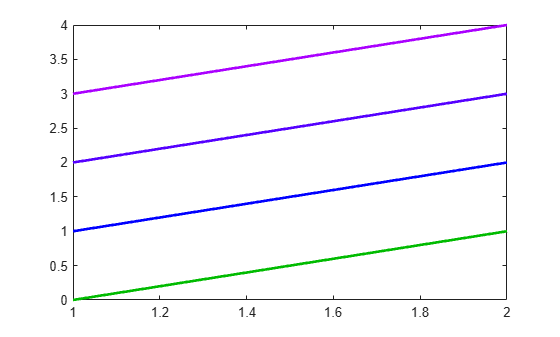You can also specify one of several named color palettes. Change the colors to the palette named `sail`.

`colororder("sail")`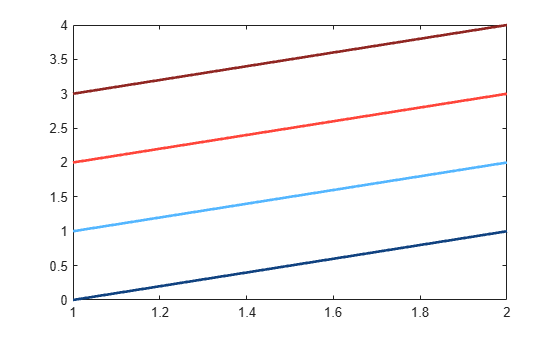Display three series of bars. Then set the color order to blue, purple, and gray.

```bar([10 20 30; 25 35 45; 30 40 52]) newcolors = [0 0.5 1; 0.5 0 1; 0.7 0.7 0.7]; colororder(newcolors)```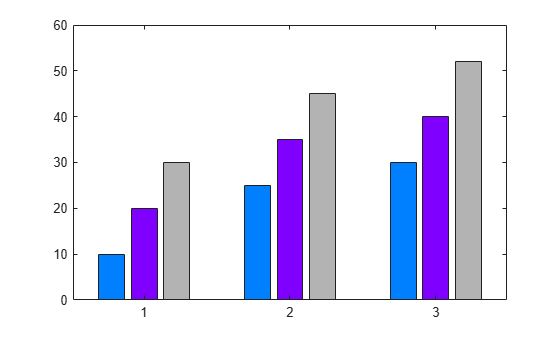Setting the color order for the figure before calling `yyaxis` sets the color for each y-axis. The left side uses the first color, and the right side uses the second color. If you specify more than two colors, the additional colors are not used by either side.

Define `newcolors` as a matrix containing two RGB triplets. Set the color order for the figure, and plot two lines against the left side. Then plot two lines against the right side.

```newcolors = [0.40 0.30 0.90; 0.50 0.65 0.15]; colororder(newcolors) % Left side yyaxis left plot([1 2; 3 4]) % Right side yyaxis right plot([4 3; 2 1])```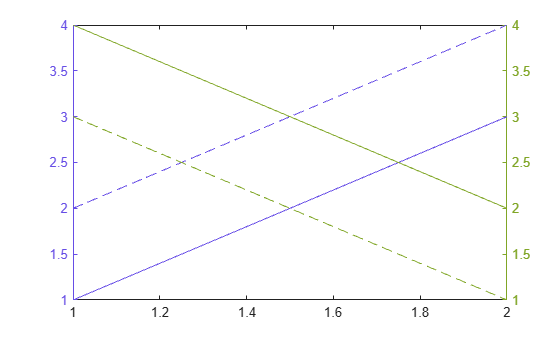Setting the color order for the figure after calling `yyaxis` sets the color for the active side.

Activate the left y-axis and plot three lines. Set the line style order to one solid line and change the y-axis color to blue. Then set the color order to three shades of blue.

```% Left side yyaxis left plot([1 2 3; 4 5 6]) ax = gca; ax.LineStyleOrder = '-'; ax.YColor = 'blue'; leftcolors = [0 0 1; 0 0.50 1; 0 0.80 1]; colororder(leftcolors)```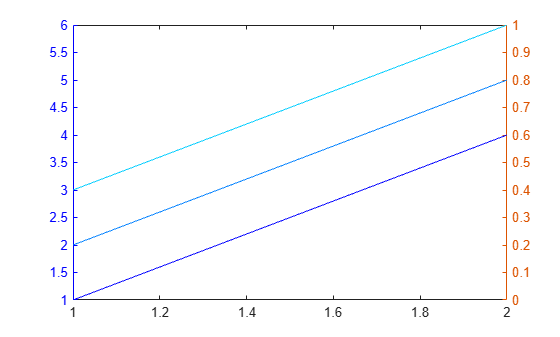Activate the right y-axis and plot two lines. Change the y-axis color to black. Then set the color order to black.

```% Right side yyaxis right plot([4 3; 2 1]) ax.YColor = 'black'; colororder('black')```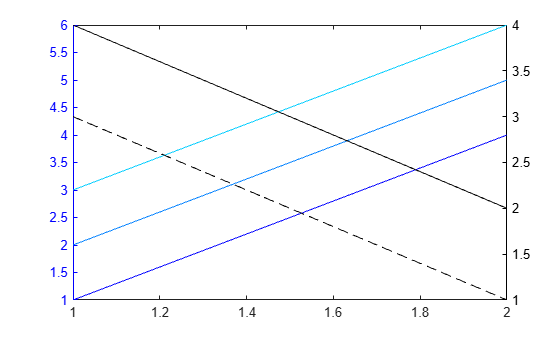When you call a plotting function with a color argument, the plotting function uses that color instead of the next color in the color order.

Set the color order of the figure to red, magenta, and blue. Call the `scatter` function to plot a series of scattered points. Then plot a second series of points, and specify the markers as black asterisks.

```newcolors = {'red','magenta','blue'}; colororder(newcolors) scatter(1:10,rand(1,10),'filled') hold on scatter(1:10,rand(1,10),'*k')```Plot a third series of points without specifying the marker color. Notice that this series uses the third color in the color order, which is blue.

```scatter(1:10,rand(1,10),'filled') hold off```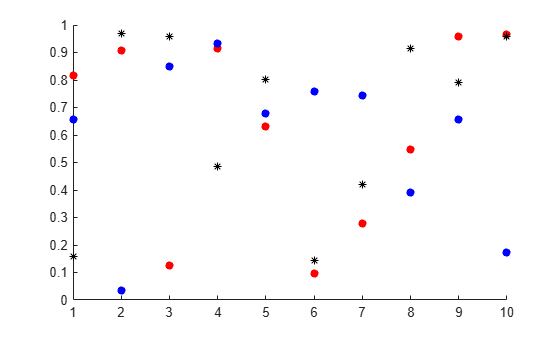Create a tiled chart layout and plot three lines in the first tile.

```tiledlayout('flow') nexttile plot([1 2 3; 4 5 6],'LineWidth',2)```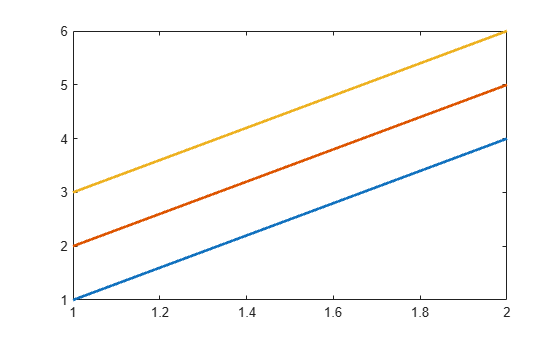Call the `nexttile` function with a return argument to get the axes object for the second tile. Plot three lines in the second tile. Then get the color order matrix for the axes and return the output in `C`. Change the first color in `C` to purple, and set the axes color order to the modified `C` matrix.

```ax = nexttile; plot(ax,[4 5 6; 1 2 3],'LineWidth',2) C = colororder(ax); C(1,:) = [0.5 0 1]; colororder(ax,C)```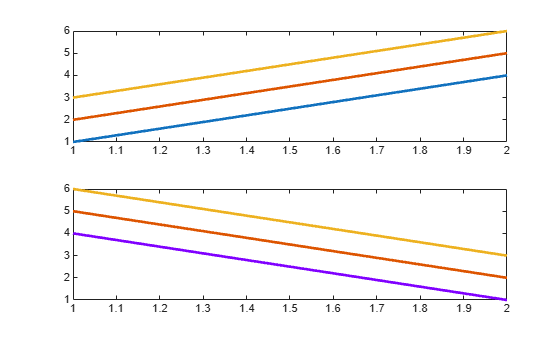Since R2023b

Named palettes provide a convenient way to change the colors of a chart. This example compares four different palettes in a tiled chart layout.

Create a tiled chart layout containing one axes object by calling the `nexttile` function. Then create a bar chart of random numbers using the default palette, `gem`.

```nexttile bar(rand(3,5)) title("gem")```Create three more bar charts using the `reef`, `meadow`, and `earth` palettes. To specify the colors for the different axes, you must pass the axes object to the `colororder` function. To get the axes objects, specify an output argument when you call `nexttile`.

```ax2 = nexttile; bar(rand(3,5)) colororder(ax2,"reef") title("reef") ax3 = nexttile; bar(rand(3,5)) colororder(ax3,"meadow") title("meadow") ax4 = nexttile; bar(rand(3,5)) colororder(ax4,"earth") title("earth")```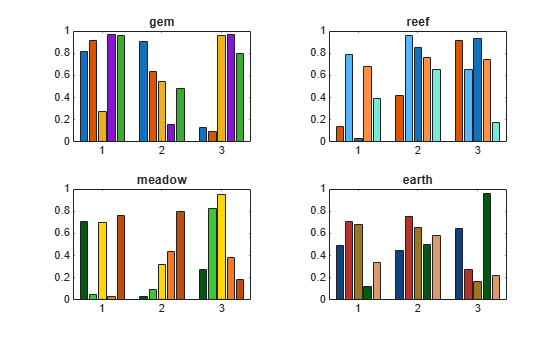## Input Arguments

collapse all

Color array, specified as a matrix of RGB triplets, an array of color names.

#### Matrix of RGB Triplets

Specify an m-by-3 matrix, where each row is an RGB triplet. An RGB triplet is a three-element vector containing the intensities of the red, green, and blue components of a color. The intensities must be in the range `[0,1]`. For example, this matrix defines the new colors as blue, dark green, and orange:

```colorarray = [1.0 0.0 0.0 0.0 0.4 0.0 1.0 0.5 0.0];```

#### Array of Color Names or Hexadecimal Color Codes

Specify any combination of color names, short names, or hexadecimal color codes.

• To specify one color, set `colorarray` to a character vector or a string scalar. For example, `colorarray = 'red'` specifies red as the only color in the color order.

• To specify multiple colors, set `colorarray` to a cell array of character vectors or a string array. For example, ```colorarray = {'red','green','blue'}``` specifies red, green, and blue as the colors.

A hexadecimal color code starts with a hash symbol (`#`) followed by three or six hexadecimal digits, which can range from `0` to `F`. The values are not case sensitive. Thus, the color codes `'#FF8800'`, `'#ff8800'`, `'#F80'`, and `'#f80'` are equivalent.

This table lists the color names and short names with the equivalent RGB triplets and hexadecimal color codes.

Color NameShort NameRGB TripletHexadecimal Color CodeAppearance
`"red"``"r"``[1 0 0]``"#FF0000"``"green"``"g"``[0 1 0]``"#00FF00"``"blue"``"b"``[0 0 1]``"#0000FF"``"cyan"` `"c"``[0 1 1]``"#00FFFF"``"magenta"``"m"``[1 0 1]``"#FF00FF"``"yellow"``"y"``[1 1 0]``"#FFFF00"``"black"``"k"``[0 0 0]``"#000000"``"white"``"w"``[1 1 1]``"#FFFFFF"`Data Types: `single` | `double` | `char` | `cell` | `string`

Since R2023b

Predefined palette name, specified as one of the values in this table or `"default"`, which is the same as `"gem"`.

Palette NamePalette Colors

`"gem"`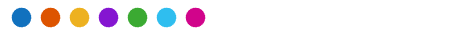`"gem12"`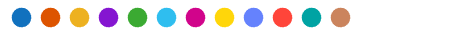`"glow"`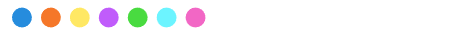`"glow12"``"sail"``"reef"`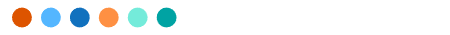`"meadow"``"dye"`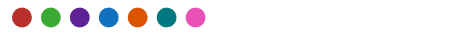`"earth"`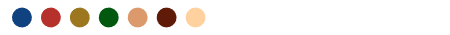Target, specified as one of these values:

## Tips

• When you set the color order for a figure, the colors persist when you call a plotting function. However, if you pass an axes object to the `colororder` function, you must first call `hold on` to make the colors persist when you call a plotting function.

• If you set the `ColorOrderIndex` or `LineStyleOrderIndex` property on the axes, the new color order does not affect existing plots. The new colors take effect only after you call ```hold on``` and then call a plotting function.

## Version History

Introduced in R2019b

expand all